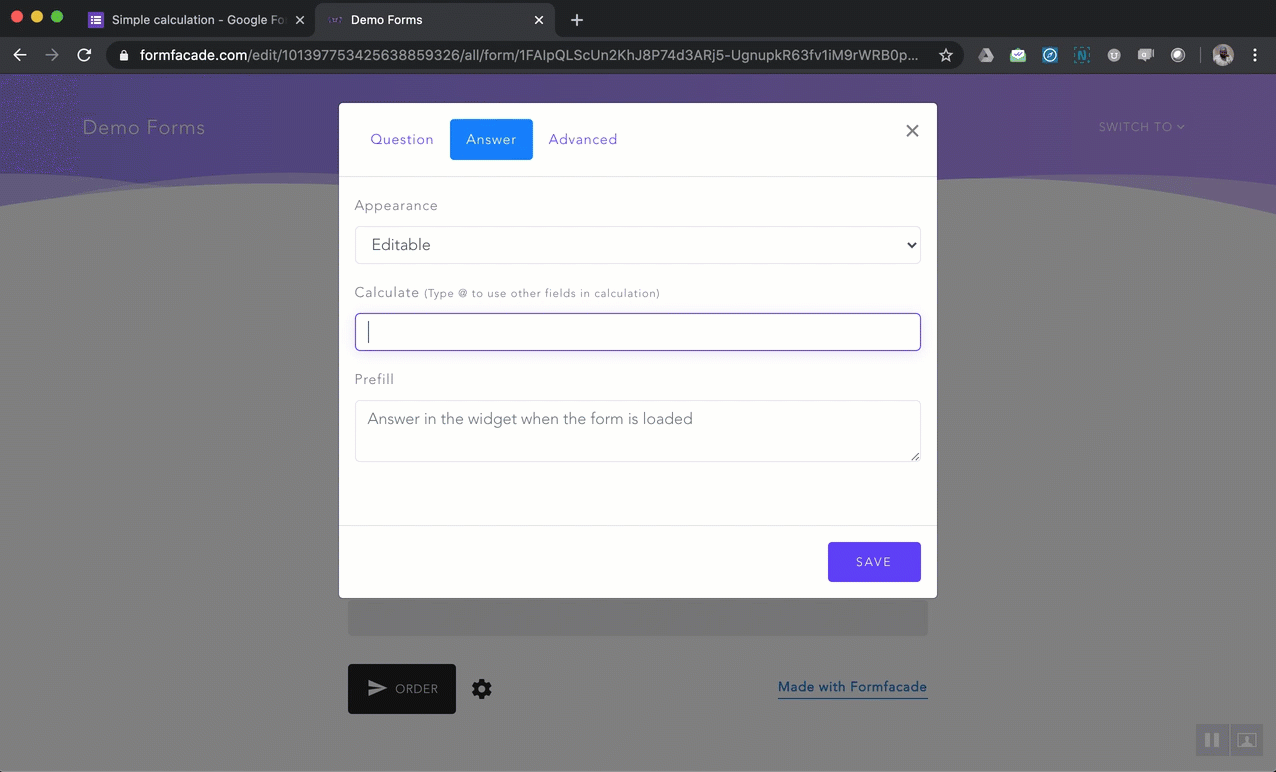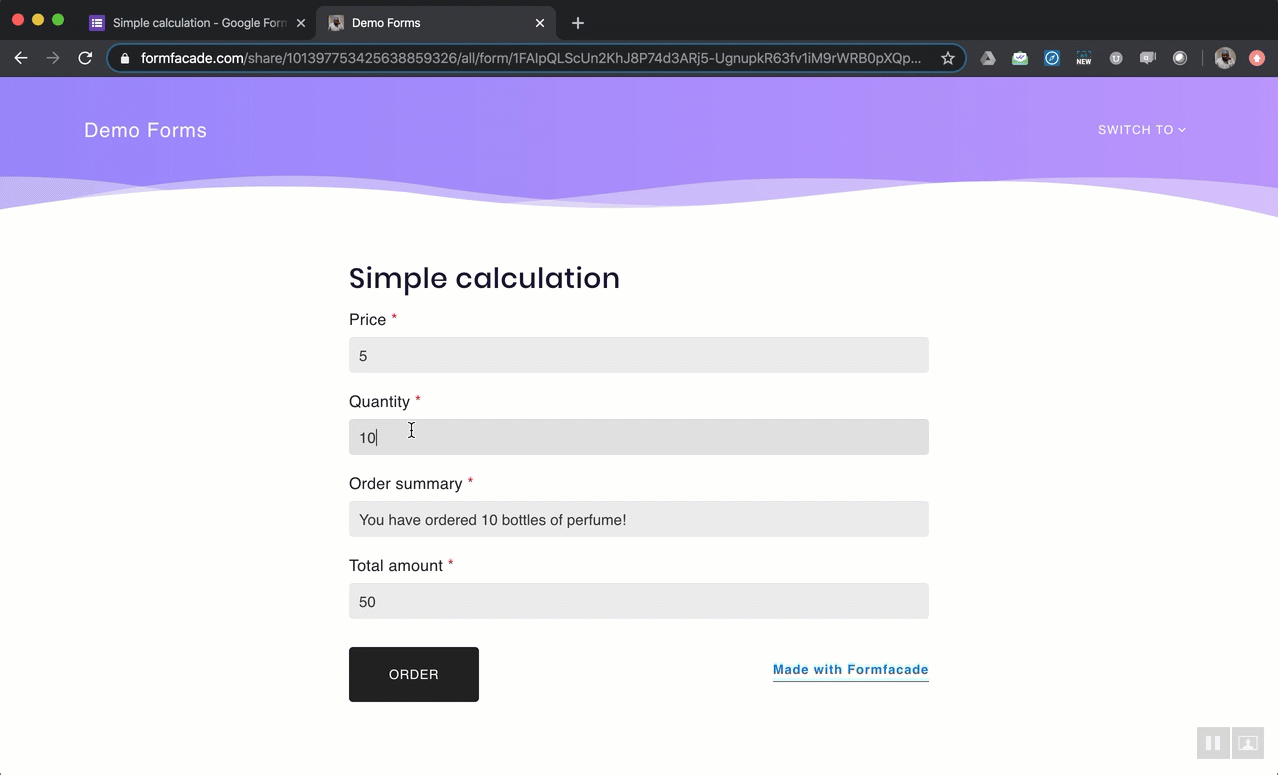# How to add a simple calculated field to a google form?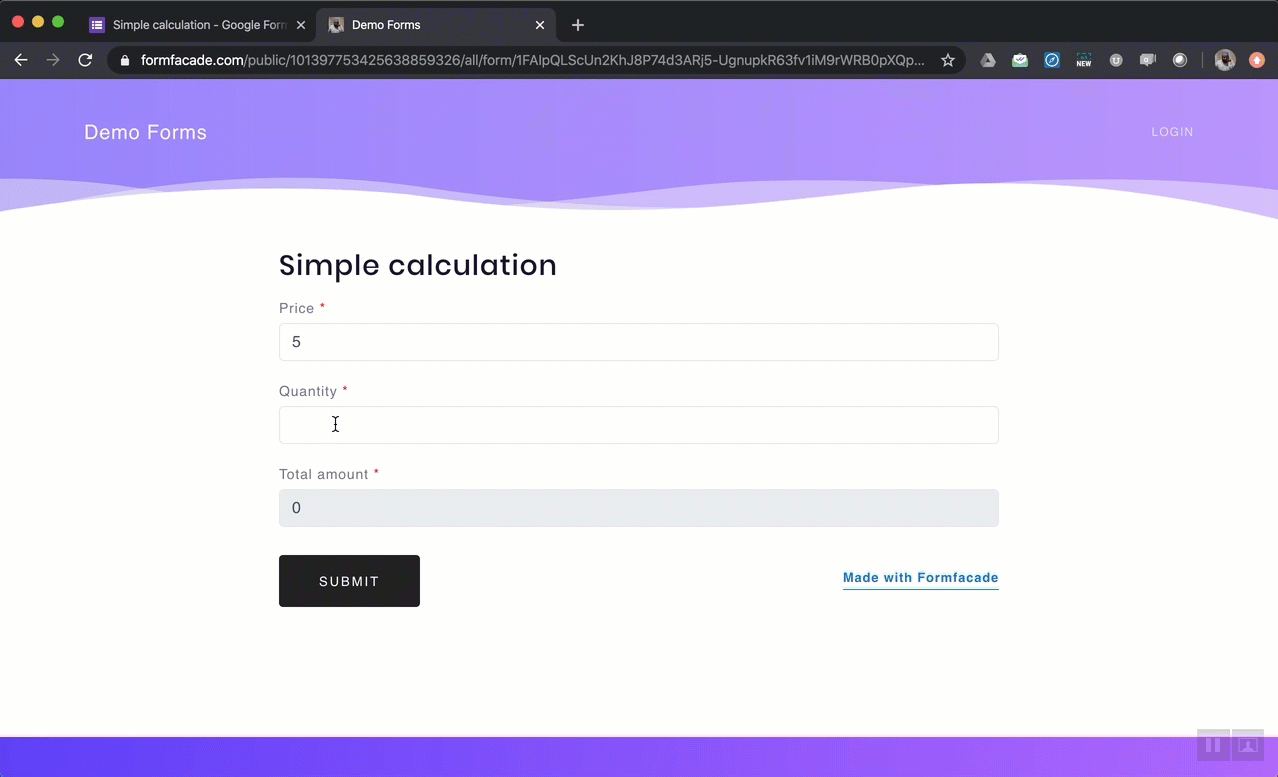Google Forms does not support dynamic calculations, but you can use Formfacade for calculating and displaying calculated values in your form. For example, you can calculate the total amount in order form based on the price and order quantity entered by the user.

#### How to show dynamic text using form data?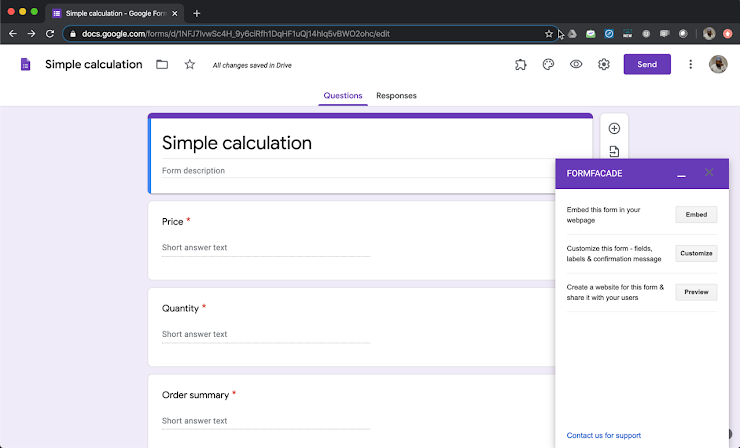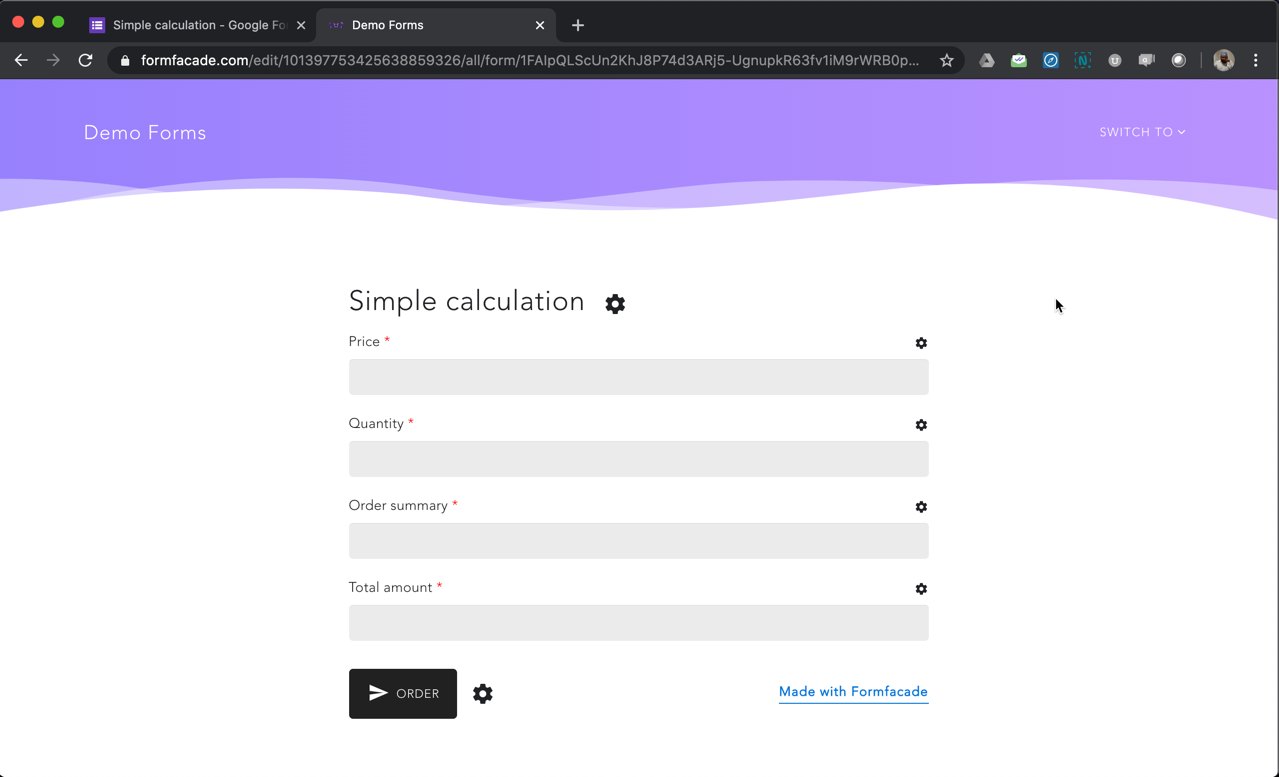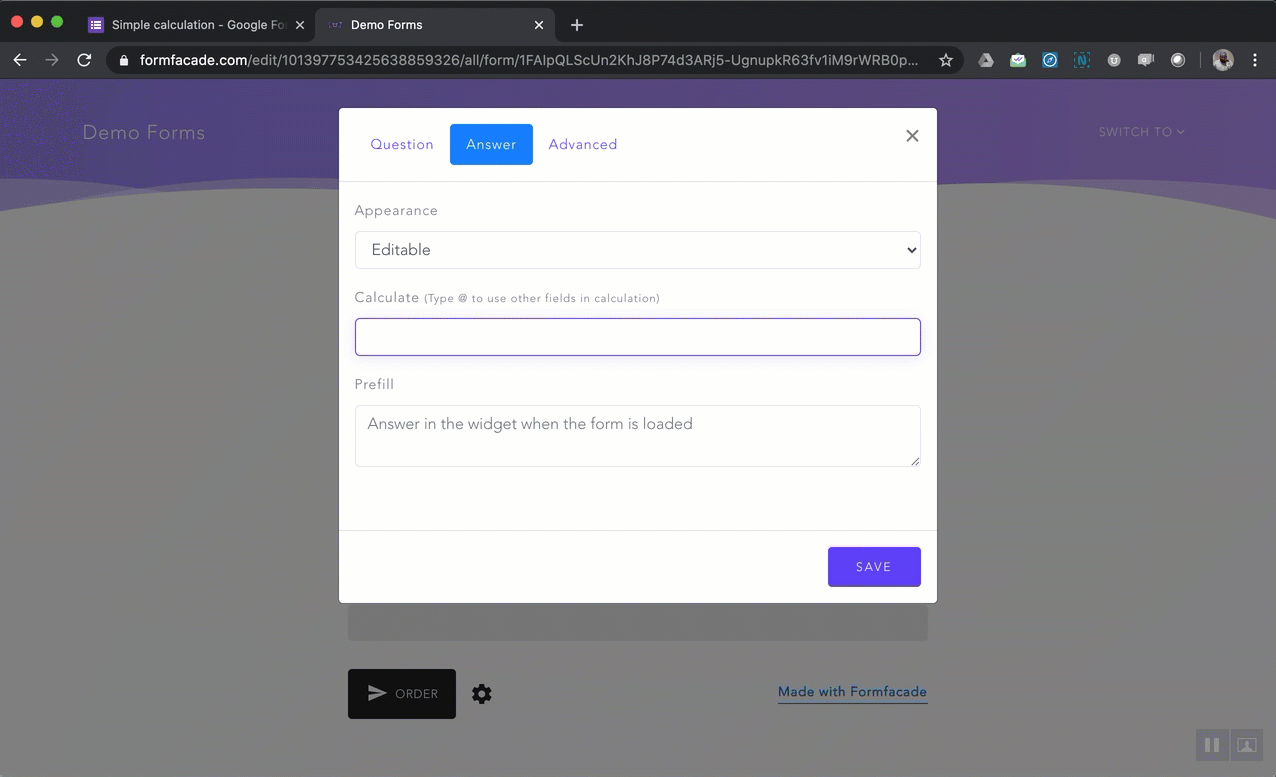#### How to do simple calculations using form data?

Let's calculate the total amount by multiplying order quantity & price form fields.

The calculations can only be performed on number fields. Add the "IsNumber" response validation for Short Answer question. Any question with IsNumber field will be automatically treated as number so that you can do calculations on those fields.